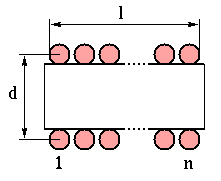# Single-layer Coil Inductance and Q

This single-layer coil inductance calculator uses the Lundin's formula  for which the maximum relative error is stated to be less than 3 ppm.
The Q value is computed here using the formula of ; the value will be just a rough estimation; moreover the effect of the coil distributed capacity are not taken into account.

## INPUT DATA

```Coil diameter,   d :  m
Coil length,     l :  m
Number of turns, n :

Frequency,     MHz :
(used only for computing Q)
```

## CALCULATED VALUES

```Inductance Value,  L :  µH

Inductor Q,        Q :

```

Most of the formulas for the inductance of a coil are valid for the current sheet approximation, where the current flows in an indefinitely thin surface around the coil diameter. This is the same as assuming the coil wound with an indefinitely thin tape with negligible separation between turns. If the separation between turns is not small, a correction factor should be applied. Moreover, at high frequencies the current crowds towards the inside of the coil so the effective radius where the current flows become smaller. Sometimes it is suggested to use the internal radius of the coil instead of the wire mean radius in the calculations, in order to compensate for this effect. However the difference between the low- and high-frequency inductances is usually not large .
To compute accurately the inductance of any kind of coil (or also of more complicated conducting structures) one has to use an electromagnetic simulator.

Regarding current sheet inductance formulas for single-layer coils, one of the most widely known is the one by Wheeler , which states (after converting to metric units):

``` L = (d2n2) / (l + 0.45d) [μH]
```

where d is the coil diameter in meters, n the number of turns and l the coil length in meters.
The above formula is accurate within 1 % for l>0.4d ; for shorter coils one can use the well-known Nagaoka formula  (which has the inconvenience of requiring a list of tabulated values for different diameter/length ratios) or other asymptotic approximations .

Some useful formulas, applicable for any diameter to length ratio, are presented in  and ;

References:

  F.E. Terman, "Radio Engineers' Handbook," London, McGraw-Hill, 1st ed., Sep. 1950.  H.A. Wheeler, "Simple Inductance Formulas for Radio Coils," Proc. I.R.E., vol. 16, pp. 1398-1400, Oct. 1928.  H. Nagaoka, "The Inductance Coefficients of Solenoids," J. Coll. Sci., vol. 27, pp. 18-33, 1909.  H.A. Wheeler, "Inductance Formulas for Circular and Square Coils," Proc. IEEE, vol. 70, no. 12, pp. 1449-1450, Dec. 1982.  R. Lundin, "A Handbook Formula for the Inductance of a Single-Layer Circular Coil," Proc. IEEE, vol. 73, no. 9, pp. 1428-1429, Sep. 1985.  F. Langford-Smith (ed.), "The Radiotron Designer's Handbook," 4th edition, Australia, Wireless Press, 1952.